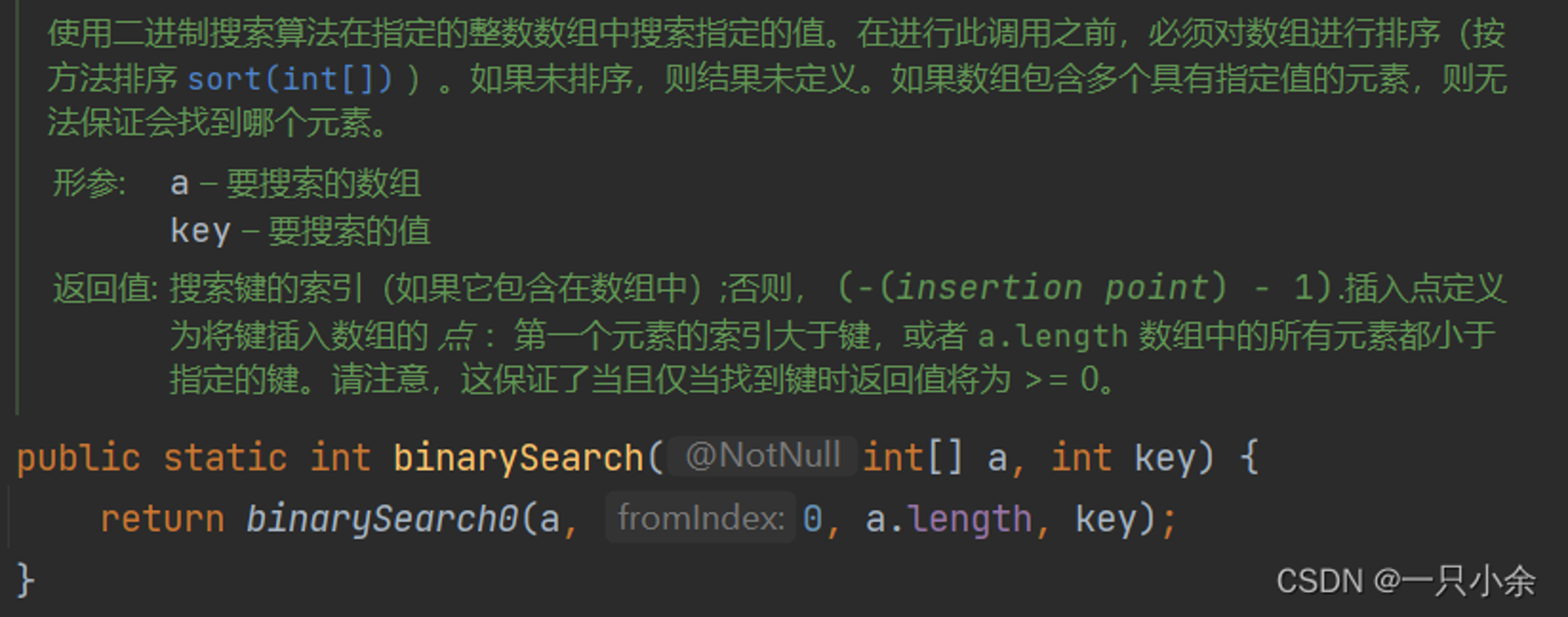V1

2023/03/04阅读：14主题：科技蓝

# 算法逻辑

1.如果比中间位置的元素大，则继续在后半部分的数列中进行二分查找；
2.如果比中间位置的元素小，则在数列的前半部分进行比较；
3.如果相等，则找到了元素的位置。

# 算法实现

## 简单实现

``// 二分查找public static int binarySearch(int[] a, int target) {    int left = 0;    int right = a.length - 1;     while (left <= right) {        int mid = (left + right) / 2;        if (a[mid] == target) {            return mid;        } else if (a[mid] < target) {            left = mid + 1;        } else {           right = mid - 1;       }   }   return -1; }``

## left+right可能超过int表示的最大限度

java中int类型占4个字节的最大值是 , 即 2147483647

（有没有可能超过32位，2个int数相加最大不会大于 ，所以一定不会超过int存储）

``int mid =(left+right)>>>2;``

## 代码分析和变换

``if (a[mid] < target) {     left = mid + 1;} else if (a[mid] > target) {    right = mid - 1;} else {   return mid;}``

``public static int binarySearch(int[] a, int target) {    int left = 0;    int right = a.length;     while (right - left <= 1) {        int mid = (left + right) / 2;        if (target < a[mid]) {            right = mid;        } else{            left = mid;        }       }       return a[i]==target?i:-1;   }   return -1; }``

## 更多需求：求索引最小的值

``public static int binarySearchMin(int[] a, int target){ int l=0,r=a.length; while(l < r){  int m = (l + r) >>> 1;  if (a[m] < target)   l = m + 1;  else   r = m; } return l;}``

``return a[l]==target?l:-(l+1);``

## java二分API

``binarySearch(数组，key)``源代码：和我们实现的差不多。``public static int binarySearch(int[] a, int fromIndex, int toIndex,int key)fromIndex – 要搜索的第一个元素（包括）的索引 toIndex – 要搜索的最后一个元素（独占）的索引``

# 应用

## 基础题

``public class Solution extends VersionControl {    public int firstBadVersion(int n) {        int l=1;int r=n;        while(l < r){            int mid=(l+r)>>>1;            if(isBadVersion(mid))                r=mid;            else                l=mid+1;        }        return l;    }}``

leetcode34. 在排序数组中查找元素的第一个和最后一个位置

``public int[] searchRange(int[] nums, int target) {        int x = -1, y = -1;        int l = 0, r = nums.length - 1;        while (l < r) {            int m = (l + r) >>> 1;            if (nums[m] >= target)                r = m;            else                l = m + 1;        }        if (l < 0 || l > nums.length-1||nums[l] != target)            return new int[]{x, y};        x = l;        r = nums.length - 1;        while (l < r) {            int m = (l + r) >>> 1;            if (nums[m] > target)                r = m - 1;            else                l = m + 1;        }        y = l - 1;        return new int[]{x, y};    }``

## 思考难度

leetcode 4. 寻找两个正序数组的中位数

1. 如果l为奇数，则中位数为(l+1)/2的位置
2. 如果为偶数，则中位数为(l+1)/2和(l+2)/2的平均数
即求指定位置k的数。

n1=[1,2,4,5,6]
n2=[2,3,5,7,9,11]
l=11 k=6 k/2=3
n1=4<n2=5

n1=[5,6]
n2[2,3,5,7,9,11]

n1=[5,6]
n2[3.5,7,9,11]

k=1

1.能不能在k=2的时候返回大值呢？

2.如果k/2大于一个数组长度呢？

``public double findMedianSortedArrays(int[] nums1, int[] nums2) {    int n = nums1.length;    int m = nums2.length;    int left = (n + m + 1) / 2;    int right = (n + m + 2) / 2;    //将偶数和奇数的情况合并，如果是奇数，会求两次同样的 k 。    return (search(nums1, 0, nums2, 0, left) + search(nums1, 0, nums2, 0, right)) * 0.5; }// a为一个数组，i为这个数组剩下的.b同理// k 为需要求的数的位置public int search(int[] a,int i,int[] b,int j,int k){ //求剩余长度 int l1 = a.length-i; int l2 = b.length-j; //调整l1永远为短的 if(l1>l2) return search(b,j,a,i,k);  if(l1 == 0) return b[j+k-1]; if (k == 1) return Math.min(a[i], b[j]); int q = k/2; //x,y 为索引 int x = i + Math.min(q,l1) - 1; int y = j + Math.min(q,l2) - 1; if(a[x] > b[y])  return search(a,i,b,y+1,k-(y-j+1)); else  return search(a,x+1,b,j,k-(x-i+1));}``

## 方法

leetcode2439. 最小化数组中的最大值

``````输入：nums = [3,7,1,6]

1. 选择 i = 1 ，nums 变为 [4,6,1,6] 。
2. 选择 i = 3 ，nums 变为 [4,6,2,5] 。
3. 选择 i = 1 ，nums 变为 [5,5,2,5] 。
nums 中最大值为 5 。无法得到比 5 更小的最大值。

``````

``````输入：nums = [10,1]

``````

1.根据题目要求右边只能降，左边只能升。第一个值可以从后面所有的值里面取过来。

2.所以对一个最大值m是否符合条件，我们可以从左往右遍历，如果这个数k比他小那么，则需要m-k个位置，如果比他大，那么把需要减掉，这时如果需求为负数，则一定不是这个数。

``public int minimizeArrayValue(int[] nums) { int l = 0,r = Integer.MAX_VALUE; while(l < r){  int m = (l+r)>>>1;  if(check(nums,m))   r = m;  else   l = m+1; } return r;}public static boolean check(int[]a,int m){ long count = 0; for(int i:a){  if(i<m)   count+=m-i;  else{   count -= i-m;   if(count < 0)   return false;  } } return true;}``V1